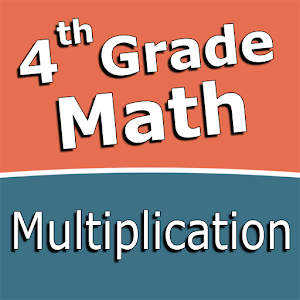English

Every day we offer FREE licensed Android apps and games you’d have to buy otherwise.\$2.99
EXPIRED

# Android Giveaway of the Day - Fourth grade Math - Multiplication

A math learning and calculation practice suite.
\$2.99 EXPIRED
User rating: 0 (0%) 0 (0%)

This giveaway offer has expired. Fourth grade Math - Multiplication is now available on the regular basis.

Intuitive interface powered by handwriting input and a fun and engaging mini game in addition to a regular math trainer mode make our app stand out from the crowd of generic math learning apps.

With Fourth grade Math - Multiplication you can practice and improve the following math skills:
- Multiplication facts up to 10×10
- Multiplication facts up to 12×12
- Multiply 1-digit numbers by 2-digit numbers
- Multiply 1-digit numbers by 3-digit numbers
- Multiply 1-digit numbers by 4-digit numbers
- Multiply 2-digit numbers by 2-digit numbers
- Multiply numbers ending in zeroes
- Multiply three numbers up to 10 each

Choose between two different modes:
- Math Trainer
- Math Shot mini-game

Math Trainer mode helps you to master the math facts, you can solve math problems at you own pace without any time constrain.

Math Shot is a math mini-game with fun and engaging game-play and as we all know learning through play and fun is more effective. The game difficulty adapts to player's skills and makes the game suitable for all ages. Math Shot helps students to develop math fact fluency - the result of consistent learning, repetition, and practice.

Sergey Malugin

Educational

2.0.0

16M

Everyone

### Compatibility:

4.0 and up